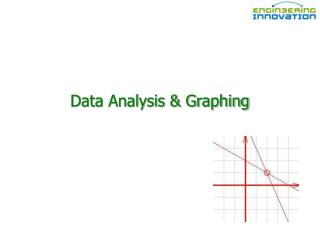DownloadDownload PresentationData Analysis & Graphing

# Data Analysis & Graphing

Télécharger la présentation## Data Analysis & Graphing

- - - - - - - - - - - - - - - - - - - - - - - - - - - E N D - - - - - - - - - - - - - - - - - - - - - - - - - - -
##### Presentation Transcript

1. Data Analysis & Graphing

2. Suppose I gave you a table of data

3. A long, long table… How does one make sense of all this data?

4. Surface temperature (last Sunday) y x The figure is capable of revealingpatternsthat are not obvious in the data!

5. Dependence on Time (last Sunday) noon 4:00 pm 9:00 am 5:00 am

6. It’s evident that… Figures can • Re-tell the data • Reveal patterns • tell a story

7. Multi-dimensional imaging Consider an enormous data table containing frequencies and the variables x, y, t,andT.

8. Wind velocity… what’smissing?

9. Velocity is a vector

10. Many types of graphs

11. Data summing to 100%...

12. Where do WIE students live?

13. Where do commuter students live (2006)? Residential Commuter

14. Graphing requirements • Axis labels • Unit designations • Title • Legend • Caption • A story

15. Scaling – telling a BAD story

16. Graphs in Labs • How did you present your data in the college rankings lab? • How did you present your data in the materials lab? • Why?

17. When is a table better than a figure? Five significant digits

18. What’s the density of a 7% mixture? The table shows that the density is 0.98347 g/cm3

19. Data Analysis: Linear Interpolation What is the density of a 5.5% ethyl alcohol solution at 25 C? Determine by linear interpolation!

20. Data Analysis: Linear Interpolation To solve, assume a linear relationship between: • Density and temperature and/or • Density and % ethyl alcohol

21. Data Analysis: Bilinear Interpolation What if there is a non-linear relationship between x and y? y x Over small Δx, can still approximate a linear relationship between x and y.

22. Data Analysis: Bilinear Interpolation To solve, assume a linear relationship between: • Density and temperature and • Density and % ethyl alcohol 5.5%, T=23C *

23. Practice Problem… then break